Problem 4:

What is the global minimum of the function?

Solution:

Look at the function for reasonably small values of x and y.

 > restart; F := (x,y) -> exp(sin(50*x)) + sin(60*exp(y)) + sin(70*sin(x)) + sin(sin(80*y)) - sin(10*(x+y)) + 1/4*(x^2+y^2);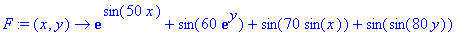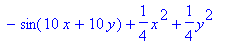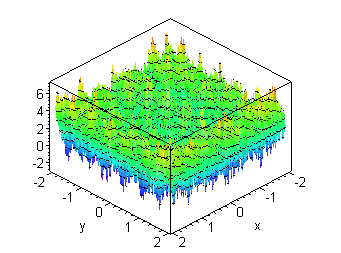It looks like a nightmare for someone trying to find the global minimum: there are huge numbers of local minima.  Note however that there's an easy lower bound: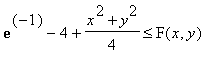.  From the graph it looks like there are points where F(x,y) is below -2.  In fact, the minimum value plotted is

 > P:= %:

 > G:= op([1,3],P);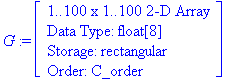> CurrMin:=min(seq(seq(G[i,j],i=1..100),j=1..100));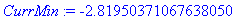For any point doing better than that,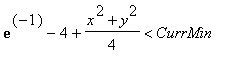so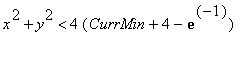> rmax:=sqrt(4*(CurrMin+4-exp(-1.0)));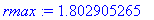So we certainly don't have to search farther away from the origin than that.  Now suppose we search in a rectangular grid such that every point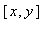in the disk of radius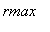in the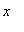direction and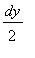in the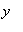direction of a grid point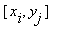.  If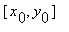is the actual minimum (so in particular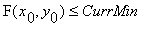), how much bigger than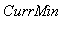can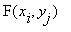be?  Well, from Taylor's Theorem (remembering that the first derivatives of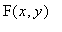at the minimum are 0),for some point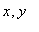on the line segment fromto.  In particular if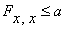,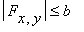and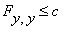, we have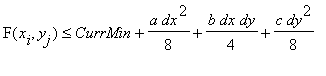.

 > a:= op([1,2],evalf(evalr(subs(x=INTERVAL(-rmax..rmax),y=INTERVAL(-rmax..rmax),diff(F(x,y),x\$2)))));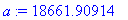> b:= op([1,2],evalf(evalr(subs(x=INTERVAL(-rmax..rmax),y=INTERVAL(-rmax..rmax),abs(diff(F(x,y),x,y))))));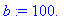> c:= op([1,2],evalf(evalr(subs(x=INTERVAL(-rmax..rmax),y=INTERVAL(-rmax..rmax),diff(F(x,y),y,y)))));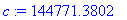With dx = .006 and dy = .002, I get

 > dz:=eval(a*dx^2/8 + b*dx*dy/4 + c*dy^2/8,{dx=.006,dy=.002});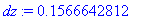> ti:= time(): dx:= .006: dy:= .002: candidates:= NULL; imax:= ceil(rmax/dx); for i from -imax to imax do   xi:= i*dx;   if abs(xi) > rmax then jmax:= 1   else jmax:= ceil(sqrt(rmax^2-xi^2)/dy)   fi;   for j from -jmax to jmax do     yj:= j*dy;     Fij:= evalhf(F(xi,yj));     if Fij < CurrMin + dz then       candidates:= candidates,[xi,yj,Fij];       if Fij < CurrMin then         CurrMin:= Fij;         bestcand:= [xi,yj];       fi     fi   od od: (time()-ti)*seconds;

 >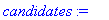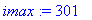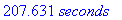> nops([candidates]);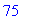> CurrMin;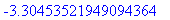We've come down quite a bit from the previous CurrMin.  Now we can search closer to each of the 75 candidates.

 > dx:= .0006; dy:= .0002; dz:= a*dx^2/8 + b*dx*dy/4 + c*dy^2/8;candidates2:= NULL: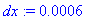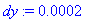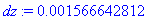> ti:= time(): for cand from 1 to 75 do   for i from -5 to 4 do     xi:= candidates[cand]+(i+1/2)*dx;     for j from -5 to 4 do       yj:= candidates[cand]+(j+1/2)*dy;       Fij:= evalhf(F(xi,yj));     if Fij < CurrMin + dz then       candidates2:= candidates2,[xi,yj,Fij];       if Fij < CurrMin then         CurrMin:= Fij;         bestcand:= [xi,yj];       fi     fi   od od od: (time()-ti)*seconds;> nops([candidates2]);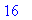> candidates2;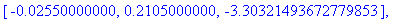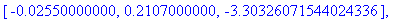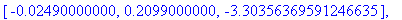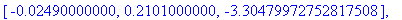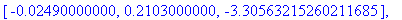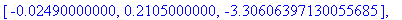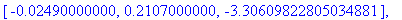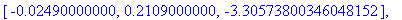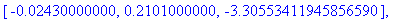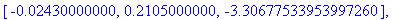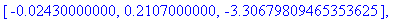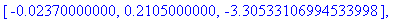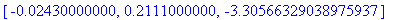> plots[display]({  plots[implicitplot](diff(F(x,y),x),x=-.0255-dx/2 .. -.0237+dx/2, y=0.2099-dy/2 .. 0.2111+dy/2, colour=red), plots[implicitplot](diff(F(x,y),y),x=-.0255-dx/2 .. -.0237+dx/2, y=0.2099-dy/2 .. 0.2111+dy/2, colour=blue) });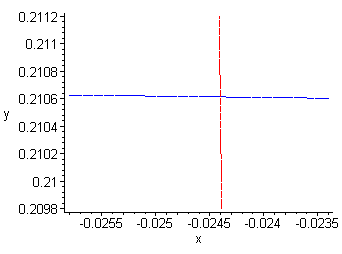> Digits:= 30; fsolve({diff(F(x,y),x), diff(F(x,y),y)},{x,y},x=-.0255-dx/2 .. -.0237+dx/2, y=0.2099-dy/2 .. 0.2111+dy/2);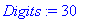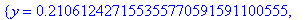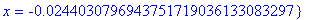> eval(F(x,y),%);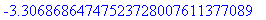All 30 digits here are correct.